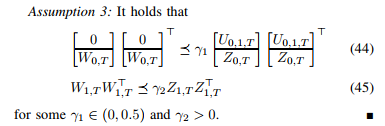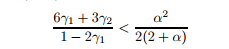# Problem in cvx form, the result is NaNHi everyone. I should resolve this problem in cvx, so I wrote in matlab:

cvx_begin sdp

variable Q(T,2)
variable a(1,1)

[Z0T*Q-a*(Z1T*Z1T') Z1T*Q; Q'*Z1T' Z0T*Q]>=eye(4)

[eye(T)  Q; Q' Z0T*Q] >= eye(9)

cvx_end


In particular:
-a is a scalar
-Q a matrix Tx2
-Z01 and Z1T matrices 2xT

The result is NaN. I have committed some mistakes?

You don’t seem to have followed my advice from your previous question Problem in cvx form . Are you solving a different problem this time? What have you done to ensure "Z0T*Qis symmetric?

It will help matters if you look at and show all CVX and solver output. Does CVX warn about nonsymmetry? Does CVX report the problem is infeasible? If you provide all the input data, forum readers can run and examine your problem.

I solved the problem with the previous question, CVX run. this second question is a sort of evolution of the first problem. I link the article with all the informations, (page 7).
http://depersis.altervista.org/papers/2019-DePersis-Tesi-preprint.pdf

maybe should I add the condition that a is define as a scalar a>0?

I warned you about the symmetry thing. It turns out that the paper’s authors also thought of that. See Remark 1 and the alternative formulation on p. 5.

ok, thanks for your help. So, should I find an alternative form of (35, how you can see from the picture of the question)?. I tried to do

cvx_begin sdp

variable Q(T,2)
variable a(1,1)

[Z0T*Q-a*(Z1T*Z1T') Z1T*Q; Q'*Z1T' Z0T*Q]>=-eye(4)

[eye(T)  Q; Q' Z0T*Q] >= -eye(9)

cvx_end


and in this case (with sign - behind function eye) I obtain the status solved.

The formulation in your post immediately above is rather boring and probably not useful for anything, as a = 0 and Q = matrix of zeros is trivially feasible.

Try the alternative formulation.mentioned in remark 1 on p. 5

I’m sorry, but I don’t understand. Why the alternative formulation.mentioned in remark 1 on p. 5 is more stable numerically?

I’m not sure that “numerical stability” (those are the authors’ words, not mine) is necessarily the best description of the difficulty. But in the original formulation, you need to address the symmetry constraint as well as making sure Q = matrix of zeros is not returned as a solution. Neither of those concerns arise in the alternative formulation. I suggest if you have additional questions on that, you directly inquire with the paper authors or post on another forum, such as https://math.stackexchange.com/ in which the merits of alternative formulations can be discussed.

thank you so mach for your help. Last question: is the following sintax corrected for the remark 1 on p.5?

cvx_begin sdp

variable Q(T,n)
variable P(n, n)

X0T*Q=P

[P  X1T*Q; Q'*X1T' P] >= eye(2*n)

cvx_end


I’m sorry, but I’m new in the use of cvx

I made 3 changes. I think that should work. If somehow the minimum eigenvalue of [P X1T*Q; Q'*X1T' P] doesn’t come out to be sufficiently positive, then perhaps change the RHS of the semidefinite constraint from 0 to something such 1e-5*eye(2*n)

   cvx_begin sdp
variable Q(T,n)
variable P(n, n) symmetric
X0T*Q == P
[P  X1T*Q; Q'*X1T' P] >= 0
cvx_end`

thanks a lot for your help, finally I solved the problem thanks to your suggestions. Now i should add an other condition to my problem:W0T, W1T, U01T, Z0T are note

I should found γ1 and γ2 in order to respect this conditionwhere alpha is the parameter a that I tried to calculated in the first part of this topic.

Is there a way to solve it?

(https://arxiv.org/pdf/1903.06842.pdf, page 9)

What exactly do you want to add? I don’t know what arr variables and what is input data - so you need to figure that out. if \gamma_1 is a variable and something else also is, then (44) is not an LMI, although you could solve LMIs for a grid of fixed values of \gamma_1.

On the last listed condition, I don;t know what the variables are. If \alpha is already known, you can multiply by the denominator of the LHS to get a linear inequality, presuming \gamma_1 is < 0.05, which makes the denominator positive.